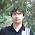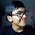## Tuesday, December 11, 2018

### when it should have returned 3.0

Let me put the weekly summaries aside for a moment and talk about the TCO 2018 finals experience, while it's still relatively fresh. As the TCO finals is one of the most important contests of the year, naturally its problems often receive deeper analysis than usual. In this post, I'd like to dive into the easy problem. The correct solution is described in the editorial, so I will focus on the incorrect ones: namely, all solutions submitted during the contest :)

I've started to have my suspicions during the contest itself, as I was implementing a rather complicated floating-point solution which had quite a bit of subtractions of possibly close numbers. However, at that point I concluded that given that it's the first problem, most likely the floating-point part checks out.

Then I saw 3 out of 6 solutions fail the system test, which was completely unexpected. Then I watched the recording of Scott's and Lewin's broadcast (which seems to be gone now :( Anyone has a link?), where Michal and another Michal mentioned the top-down solution, and told that the bottom-up solution that operates in formal piecewise-linear functions is very tricky to get right — but it was exactly the solution that all contestants seemed to implement.

The final realization came when I was looking at Um_nik's code for the problem, to understand why it could fail the system tests. It all seemed to check out, but had one big difference with, say, my own code: it used 64-bit integers to represent the x-coordinates of the junction points of the piecewise-linear function instead of doubles. It is true that all junctions have integer x-coordinates, so this strongly pointed to the fact that 64-bit integers simply overflow, which was indeed the issue.

But then it occurred to me: if 64-bit integers overflow, and the answer can be very small, how come the double-based solutions have enough precision? Armed with the knowledge that super large x-coordinates are possible, I've taken another look at my solution, and the potentially problematic line immediately stood out:

`return value[pos] + slope[pos] * (at - control[pos]);`

Here I'm trying to evaluate a linear function when x=at. There are actually two subtractions in this line: one denoted with a minus, and one with a plus :)

The at - control[pos] one is actually not an issue by itself, as both at and control[pos] are integers, and the best answer is always found for relatively small values of at, so when control[pos] is also small this subtraction is exact, and when it's big we're not subtracting two close numbers anymore.

However, the plus is actually an issue. Suppose at is small, the value of the linear function there is also small, but both control[pos] and value[pos] are big, then we're subtracting one huge number (-slope[pos] * (at - control[pos])) from another (value[pos]) to get a tiny result, and this is where the small relative error turns into a huge absolute error.

In order to construct a concrete testcase, we need to understand how to get a segment in our piecewise-linear function such that one end has both coordinates small, and the other end has both coordinates big. We can notice that if we take any rooted tree and its corresponding piecewise-linear function, and add a new root which has two children, the old tree and a new leaf with value 0, then what happens is that the x-coordinates of all junction points get multiplied by 2, and the y-coordinates of the junction points get the absolute value of corresponding x-coordinate added to them.

Let's start with a tree with a root and two leaves, with values -1 and 1. Its piecewise-linear function has junction points (-2,2) and (2,2). Now we grow it using the above trick n times, obtaining a caterpillar (see the picture on the right), with the piecewise-linear function defined by the junction points (-2n+1,2n+1), (0,2) and (2n+1,2n+1). Everything so far is computed exactly right as powers of two are representable in double.

Now we do one last caterpillar expansion step, but the new leaf has value of either 1 or -1, depending on which exact bug we're trying to exploit. This causes the solution to evaluate the above linear function in point -1 or 1. If it uses the right boundary of the segment to do the interpolation, then having value 1 in the new leaf will cause it to subtract roughly speaking 2n+1-3 from 2n+1, which is bound to return 0 instead of 3 when n is big enough. Similarly, if a solution interpolates using the left boundary, then -1 will do the trick.

And sure enough:
Petr: The method returned 0.0 when it should have returned 3.0
tourist: The method returned 1.57412216096E21 when it should have returned 3.0
ACRush: The method returned 0.0 when it should have returned 3.0

In other words, all submitted solutions were incorrect, and the judges were completely right in saying that the piecewise-linear approach is super tricky to get right. Luckily, even if the system tests caught this, it would have no bearing on the top 4 in the standings.

Having identified the problematic line, and having understood the mechanics of the huge testcases, it's now relatively easy to come up with a fix: we just need to make sure that the plus in that line is always an addition, and never a subtraction. This is achieved by using the smaller of the two y-coordinates of endpoints of the segment for interpolation, or practically by changing the if condition from

```if (pos < control.length) {
return value[pos] + slope[pos] * (at - control[pos]);
} else {
return value[pos - 1] + slope[pos] * (at - control[pos - 1]);
}```

into

```if (slope[pos] < 0) {
return value[pos] + slope[pos] * (at - control[pos]);
} else {
return value[pos - 1] + slope[pos] * (at - control[pos - 1]);
}```

in my solution. I'm reasonably certain the solution is correct after this fix, but feel free to prove me wrong and come up with another challenge case :)

Note that there's one more small thing my solution does right from precision point of view: I'm keeping some redundant information in my representation of a piecewise-linear function, storing ~3n values for a function with ~2n degrees of freedom. This allows me to evaluate each segment independently, and thus not care about precision errors on huge values which have no bearing on the final answer which is always achieved in a relatively small point. Had I for example kept the entire control, slope, but only the left-most element of value, and used running sums to get the rest, I would get precision issues even with the above fix as the low y-coordinates would already be computed incorrectly. It is possible to have only ~2n values without precision issues (i.e. keep only value and control, and the slope only before first and after last control point), but choosing such representation correctly requires some understanding of the precision issues, while having some redundancy allows to avoid more potentially problematic computations.

Thanks for reading, and check back for more weekly summaries as I still hope to catch up with 2018 in 2018!

1.1.2.